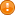# sci2exp

returns a string able to generate a given Scilab object

### Syntax

```t = sci2exp(a)
t = sci2exp(a, LHSname)
t = sci2exp(a, LHSname, lmax)
t = sci2exp(a, lmax)```

### Arguments

a

a scilab object. The supported types are:

• booleans
• encoded integers of any inttype
• real or complex numbers
• sparse matrices
• polynomials
• strings, that may include some NewLine (ascii(10)) sequences
• a structures array or cells array with any number of dimensions
• a simple list whose components types are supported by `sci2exp`
• a typed list tlist() and mlist()
• a single graphic handle (only of figure or uicontrol).

For booleans, encoded integers, real or complex numbers, polynomials and strings, any of a single element or a vector or a matrix or an hypermatrix is accepted.

LHSname

Optional string: The name of the variable building the assignment prefix `LHSname+" = "`. When it is provided, `LHSname+" = "` is prepended to the raw expression yielded from the `a` object. Then, the result string `t` can no longer be evaluated with `evstr()` but with `execstr()`.

t

Column of strings. A single whole string is returned when `lmax` is not used or is set to 0: The Scilab literal expression, with possibly the left-hand affectation part on the first line when `LHSname` is provided.

lmax

Positive integer setting the maximal length of the `t` components. The default value `0` ignores such a constrain and returns `t` as a single (long) string. Otherwise, some continuation marks `..` are used to break too long rows.Since continuation marks `..` prevent using `evstr()` and `execstr()`, using this `lmax` option mostly cancels the main `sci2exp()` purpose.

### Description

"sci2exp" stands for the conversion of a scilab object into a literal executable expression.Literal values of decimal real or complex numbers or coefficients are output in `t` according to the current format setting. So, they may be truncated with respect to the full numerical accuracy. When `a` are polynomials, the resulting expression `t` can be executed to regenerate `a` ONLY if the formal variable of the polynomials is defined in the current scope: For polynomials in `s` or `z`, the predefined Scilab variables `%s` or `%z` are used, and the regeneration can be straightforward: ```--> p = [1+%z 1-%z^2]; --> tp = pol2str(p) tp = !1+z 1-z^2 ! --> evstr(tp) at line 1 of executed string at line 38 of function evstr ( SCI\modules\string\macros\evstr.sci line 52 ) Undefined variable: z --> tp = sci2exp(p) tp = [1+%z,1-%z^2] <<< sci2exp() uses the variable %z as predefined "z" generator --> t = evstr(tp) // .. straigntforward regeneration t = 2 1 +z 1 -z --> typeof(t) ans = polynomial ``` Otherwise: The formal variable of `a` -- say `"x"` -- must be explicitly defined with (say) `x = poly(0,"x")`: ```--> p = poly([1 2 3], "x") p = 2 3 -6 +11x -6x +x --> tp = sci2exp(p) tp = -6+11*x-6*x^2+x^3 --> // Evaluating tp now would yield an error: x is not yet defined. We must prioly do: --> x = poly(0,"x") // Defines the symbolic variable "x" in the actual variable with the SAME name x = x --> // Then evaluating tp to regenerate p is OK: --> rp = evstr(tp) rp = 2 3 -6 +11x -6x +x --> rp==p ans = T ``` When `a` is a graphic handle, `sci2exp` ignores its parent (empty) and its children.

### Examples

With a numerical matrix:

```a = [%i 2 ; 3 4-2*%i]
sci2exp(a)
sci2exp(a, 'aa')
sci2exp(a, 'aa', 10)```
```--> a = [%i 2 ; 3 4-2*%i]
a  =
i      2.
3.     4. - 2.i

--> sci2exp(a)
ans  =
[%i,2;3,4-%i*2]

--> sci2exp(a, 'aa')
ans  =
aa = [%i,2;3,4-%i*2]

--> sci2exp(a, 'aa', 10)
ans  =
!aa = [     !
!%i,2;      !
!3,4-%i*2]  !
```

With some other types of objects:

```sci2exp(ssrand(2,2,2))                      // a typed list created with tlist()

sci2exp(figure("figure_name","figure1"))    // a graphic handle```

Impact of format() on literal output numbers:

```p = [0.123456789, %z-%pi*%i];
pol2str(p)                      // also impacted by format()
format(20);
Lp = sci2exp(p)                 // Literal encoding
Rp = evstr(Lp); pol2str(Rp)     // Regenerated from literal
Rp == p
format(10);                     // Let's truncate the literal output before calling sci2exp()
Lp = sci2exp(p)
Rp = evstr(Lp); format(20); pol2str(Rp)
Rp == p```
```--> p = [0.123456789, %z-%pi*%i];

--> pol2str(p)
ans  =
!0.1234568  -%i*3.1415927+z  !

--> format(20)

--> Lp = sci2exp(p)
Lp  =
[0.12345678900000000,-%i*3.14159265358979310+%z]

--> Rp = evstr(Lp); pol2str(Rp)
ans  =
!0.12345678900000000  -%i*3.14159265358979310+z  !

--> Rp == p
ans  =
T T

--> format(10)          // Let's truncate the literal output before calling sci2exp()

--> Lp = sci2exp(p)
Lp  =
[0.1234568,-%i*3.1415927+%z]

--> Rp = evstr(Lp); format(20); pol2str(Rp)
ans  =
!0.12345680000000001  -%i*3.14159269999999990+z  !

--> Rp == p
ans  =
F F
```

• string — 文字列に変換
• prettyprint — Converts a Scilab object into some corresponding LaTeX, TeX, MathML or HTML strings
• format — set the default output format of decimal numbers
• pol2str — 多項式を文字列に変換
• evstr — 式を評価する
• execstr — 文字列中のScilabコードを実行

### History

 バージョン 記述 6.0.2 The name of the predefined variables `%s` and `%z` is now used as literal generator for input polynomials in `s` or `z`.
 Report an issue << regexp Strings strcat >>